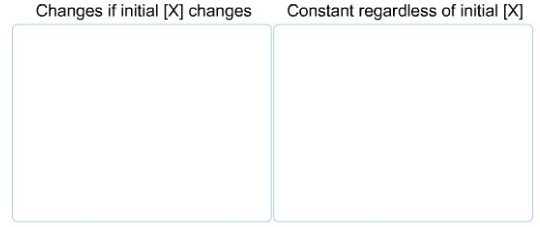# Problem: A generic gas, X, is placed in a sealed glass jar and decomposes to from gaseous Y and solid Z.2X(g) ⇌ Y(g) + Z(s)How are these equilibrium quantities affected by the initial amount of X(g) placed in the container? Assume constant temperature. mass of Z at equilibrium[Y] at equilibrium[Y]/[X] at equilibrium[X] at equilibrium[X]2/[Y] at equilibriumtotal pressure at equilibrium

###### FREE Expert Solution

To determine how given equilibrium quantities will be affected by the initial amount of X(g) placed in the container at constant temperature.

Reaction: 2X(g)  Y(g) + Z(s)

reactant    product

According to Le Chatelier’s Principle, if a system (chemical reaction) is at equilibrium and we disturb it, then the system will readjust to maintain its equilibrium state.

Changes in reactant concentration:

• ↑ reactants → shifts equilibrium to right → to form more products (minimize excess reactant)
• ↓ reactants → shifts equilibrium to left → to form more reactants (replenish depleted reactant)###### Problem Details

A generic gas, X, is placed in a sealed glass jar and decomposes to from gaseous Y and solid Z.

2X(g) ⇌ Y(g) + Z(s)

How are these equilibrium quantities affected by the initial amount of X(g) placed in the container? Assume constant temperature.mass of Z at equilibrium

[Y] at equilibrium

[Y]/[X] at equilibrium

[X] at equilibrium

[X]2/[Y] at equilibrium

total pressure at equilibrium